• 压缩传感重构算法包括贪婪算法，凸优化算法等。本文档介绍关于信号重构贪婪算法中的stomp算法，
• 压缩感知重构算法——FPC前言Fixed PointContinuationFPC参考 前言 所谓FPC即Fixed Point Continuation，其中涉及到两个部分，即Fixed Point和Continuation。不动点迭代算法和连续算法。下面针对这两个方面对FPC算法...


压缩感知重构算法——FPC
前言Fixed PointContinuationFPC参考

前言
所谓FPC即Fixed Point Continuation，其中涉及到两个部分，即Fixed Point和Continuation。不动点迭代算法和连续算法。下面针对这两个方面对FPC算法的原理进行阐述。
Fixed Point
首先，FPC算法是2007年Elaine T. Hale等人提出的一种解决压缩感知中L1范数最小化问题的重构算法。属于凸优化算法的一种。其解决问题的数学模型如下：目标即求minφ(x)的解x*。而我们知道，求一个函数的最优解，即求其导数的零解，即T(x)=∂φ(x)=0的解x*。 求函数T(x)=0，可以使用不动点迭代的方法，即将T(x)=0变成x=g(x)的形式，进行迭代求解。根据这个思想，我们再来看原始的目标函数φ(x),通常φ(x)可以分解为两个凸函数的相加，即φ(x)=φ1+φ2。那么我们对应的算子T也可以转换为两个单调算子的和，即T=T1+T2。之后我们开始进行不动点迭代的转换，即求T的零解。 在转换过程中我们假设参数τ>0，且T2是一个单值函数，且（I+τT1）可逆。可推出φ(x)最小解的迭代式：对于解决的凸优化问题，即T1=▽||x||1，T2= μ▽||Ax-b||22/2。同时要求（I+τT1）是收缩的。 之后针对凸优化问题的解，原论文中给出命题：其中g(x)=▽f(x)=▽μ||Ax-b||22/2。 上式（15）其中包含两个步骤，一个是梯度下降步，一个是阈值收缩步。 即最终可表示为迭代式其中v=τ/μ. 以上便是FPC的FP不动点迭代部分，
Continuation
而对于连续算法，又名同伦法。其目的是用于选择合适的μ值。 同伦法的思想即为了解决一个问题f(x)，可以构造一个新的问题H(x,s)=sf(x)+(1-s)g(x)。其中当s=1时，H(x,1)=f(x),即原问题；当s=0时，H(x,0)=g(x)。其中g(x)一般可设置为一个已知解的问题，如f(x)-f(x*)，解为x*。然后s从0到1缓慢变换，得到一个路径，这个H(x,s)即连接f(x)到g(x)的路径。然后将其融入到其他算法中，进行迭代，往往会比给定一个具体的初值的效果要更好。 有关同伦法具体解释可参考这篇博客同伦法（Homotopy Method）
FPC
而FPC算法即在不动点迭代中加入同伦法的思想，不以直接设定的初值μ来进行迭代计算，而是生成一个μ的序列，然后在这个序列中缓慢变化选择，进行迭代。下面给出FPC算法的实现步骤：参考
【1】FPC论文 【2】同伦法
展开全文• 压缩传感重构算法的matlab实现，包含pdf文档的算法描述，对于学习压缩传感理论有很大帮助。
• 压缩传感理论与重构算法。仅供学习使用，希望对大家有帮助。
• 为了能够得到更好的压缩传感重构效果, 把流形学习的思想和方法与压缩传感相结合, 提出了一种基于参数化流形学习的压缩传感重构方法。实验结果表明, 提出的方法对自然图像进行重构取得了很好的效果, 充分验证了基于...
• 压缩传感的四种重构算法，OMP算法 BP算法 分块OMP算法 StOMP算法，不是本人编写，但是经过的我的修改，希望对大家有用，很感恩这是一个免费平台，大家共同学习
• 针对传统算法在处理传感器网络的大规模信号时, 运算复杂度显著增大, 性能急剧下降的问题, 提出了启发式同步自适应迭代阈值重构算法。采用启发式差错控制函数选择代价最少的方向逐行同步收缩逼近最优解, 并结合由...传感器网络
• 为了能够得到更好的压缩传感重构效果, 把流形学习的思想和方法与压缩传感相结合, 提出了一种基于参数化流形学习的压缩传感重构方法。实验结果表明, 提出的方法对自然图像进行重构取得了很好的效果, 充分验证了基于...
• 压缩感知重构算法之OMP算法python实现 压缩感知重构算法之CoSaMP算法python实现 压缩感知重构算法之SP算法python实现 压缩感知重构算法之IHT算法python实现 压缩感知重构算法之OLS算法python实现 压缩感知重构...
压缩感知重构算法之OMP算法python实现  压缩感知重构算法之CoSaMP算法python实现  压缩感知重构算法之SP算法python实现  压缩感知重构算法之IHT算法python实现  压缩感知重构算法之OLS算法python实现  压缩感知重构算法之IRLS算法python实现
本文主要简单介绍了利用python代码实现压缩感知的过程。
压缩感知简介
【具体可以参考这篇文章】  假设一维信号

x
$x$长度为N，稀疏度为K。Φ$\Phi$ 为大小

M×N
$M\times N$矩阵

(M<<N)
$(M\lt \lt N)$。

y=Φ×x
$y=\Phi \times x$为长度M的一维测量值。压缩感知问题就是已知测量值

y
$y$和测量矩阵Φ$\Phi$的基础上，求解欠定方程组

y=Φ×x
$y=\Phi \times x$得到原信号

x
$x$。Φ的每一行可以看作是一个传感器（Sensor），它与信号相乘，采样了信号的一部分信息。而这一部分信息足以代表原信号，并能找到一个算法来高概率恢复原信号。 一般的自然信号x本身并不是稀疏的，需要在某种稀疏基上进行稀疏表示x=ψs$x=\psi s$,

ψ
$\psi$为稀疏基矩阵，

S
$S$为稀疏系数。所以整个压缩感知过程可以描述为y=Φx=ΦΨs=Θsy=Φx=ΦΨs=Θs
重建算法：OMP算法简析
OMP算法  输 入:测量值y、传感矩阵

Phi=Φψ
$Phi=\Phi \psi$、稀疏度K  初始化:初始残差 r0=y,迭代次数t=1,索引值集合index;  步 骤:  1、找到残差r和传感矩阵的列积中最大值对应下标，也就是找到二者内积绝对值最大的一个元素对应的下标，保存到index当中  2、利用index从传感矩阵中找到，新的索引集

Phit
$Phi_t$  3、利用最小二乘法处理新的索引集和y得到新的近似值

θ=argmin||y−Phitθ||2
$\theta=argmin||y-Phi_t\theta||_2$  4、计算新的残差

rt=y−Phitθ
$r_t=y-Phi_t\theta$，t=t+1  5、残差是否小于设定值，小于的话 退出循环，不小于的话再判断t>K是否成立，满足即停止迭代，否则重新回到步骤1，继续执行该算法。  输 出:θ的K-稀疏近似值

实验
要利用python实现，电脑必须安装以下程序
python （本文用的python版本为3.5.1）numpy python包（本文用的版本为1.10.4）scipy python包（本文用的版本为0.17.0）pillow python包（本文用的版本为3.1.1）
python代码
#coding:utf-8
#%%%%%%%%%%%%%%%%%%%%%%%%%%%%%%%%%%%%%%%%%%%%%%%%%%
# DCT基作为稀疏基，重建算法为OMP算法 ，图像按列进行处理
# 参考文献: 任晓馨. 压缩感知贪婪匹配追踪类重建算法研究[D].
#北京交通大学, 2012.
#
#%%%%%%%%%%%%%%%%%%%%%%%%%%%%%%%%%%%%%%%%%%%%%%%%%%%%
# 导入所需的第三方库文件
import  numpy as np
import math
from PIL import Image

#读取图像，并变成numpy类型的 array
im = np.array(Image.open('lena.bmp')) #图片大小256*256

#生成高斯随机测量矩阵
sampleRate=0.7  #采样率
Phi=np.random.randn(256*sampleRate,256)

#生成稀疏基DCT矩阵
mat_dct_1d=np.zeros((256,256))
v=range(256)
for k in range(0,256):
dct_1d=np.cos(np.dot(v,k*math.pi/256))
if k>0:
dct_1d=dct_1d-np.mean(dct_1d)
mat_dct_1d[:,k]=dct_1d/np.linalg.norm(dct_1d)

#随机测量
img_cs_1d=np.dot(Phi,im)

#OMP算法函数
def cs_omp(y,D):
L=math.floor(3*(y.shape)/4)
residual=y  #初始化残差
index=np.zeros((L),dtype=int)
for i in range(L):
index[i]= -1
result=np.zeros((256))
for j in range(L):  #迭代次数
product=np.fabs(np.dot(D.T,residual))
pos=np.argmax(product)  #最大投影系数对应的位置
index[j]=pos
my=np.linalg.pinv(D[:,index>=0]) #最小二乘,看参考文献1
a=np.dot(my,y) #最小二乘,看参考文献1
residual=y-np.dot(D[:,index>=0],a)
result[index>=0]=a
return  result

#重建
sparse_rec_1d=np.zeros((256,256))   # 初始化稀疏系数矩阵
Theta_1d=np.dot(Phi,mat_dct_1d)   #测量矩阵乘上基矩阵
for i in range(256):
print('正在重建第',i,'列。')
column_rec=cs_omp(img_cs_1d[:,i],Theta_1d) #利用OMP算法计算稀疏系数
sparse_rec_1d[:,i]=column_rec;
img_rec=np.dot(mat_dct_1d,sparse_rec_1d)          #稀疏系数乘上基矩阵

#显示重建后的图片
image2=Image.fromarray(img_rec)
image2.show()
matlab代码
%这个代码是网上某位大哥写的，在此谢过了~
function Demo_CS_OMP()
%------------ read in the image --------------
img=double(img);
[height,width]=size(img);
%------------ form the measurement matrix and base matrix -------
Phi=randn(floor(0.7*height),width);  % only keep one third of the original data
Phi = Phi./repmat(sqrt(sum(Phi.^2,1)),[floor(0.7*height),1]); % normalize each column

mat_dct_1d=zeros(256,256);  % building the DCT basis (corresponding to each column)
for k=0:1:255
dct_1d=cos([0:1:255]'*k*pi/256);
if k>0
dct_1d=dct_1d-mean(dct_1d);
end;
mat_dct_1d(:,k+1)=dct_1d/norm(dct_1d);
end

%--------- projection ---------
img_cs_1d=Phi*img;

%-------- recover using omp ------------
sparse_rec_1d=zeros(height,width);
Theta_1d=Phi*mat_dct_1d;%测量矩阵乘上基矩阵
for i=1:width
column_rec=cs_omp(img_cs_1d(:,i),Theta_1d,height);
sparse_rec_1d(:,i)=column_rec'; %  稀疏系数
end
img_rec_1d=mat_dct_1d*sparse_rec_1d;  %稀疏系数乘上基矩阵

%------------ show the results --------------------
figure(1)
subplot(2,2,1),imshow(uint8(img)),title('original image')
subplot(2,2,2),imagesc(Phi),title('measurement mat')
subplot(2,2,3),imagesc(mat_dct_1d),title('1d dct mat')
psnr = 20*log10(255/sqrt(mean((img(:)-img_rec_1d(:)).^2)));
subplot(2,2,4),imshow(uint8(img_rec_1d));
title(strcat('PSNR=',num2str(psnr),'dB'));

%*******************************************************%
function hat_x=cs_omp(y,T_Mat,m)
% y=T_Mat*x, T_Mat is n-by-m
% y - measurements
% T_Mat - combination of random matrix and sparse representation basis
% m - size of the original signal
% the sparsity is length(y)/4

n=length(y);
s=floor(3*n/4); %  测量值维数
hat_x=zeros(1,m); %  待重构的谱域(变换域)向量
Aug_t=[];        %  增量矩阵(初始值为空矩阵)
r_n=y;  %  残差值

for times=1:s; %  迭代次数(稀疏度是测量的1/4)

product=abs(T_Mat'*r_n);
[val,pos]=max(product);   %最大投影系数对应的位置
Aug_t=[Aug_t,T_Mat(:,pos)];   %矩阵扩充
T_Mat(:,pos)=zeros(n,1); %选中的列置零
aug_x=(Aug_t'*Aug_t)^(-1)*Aug_t'*y;  % 最小二乘,看参考文献1
r_n=y-Aug_t*aug_x;   %残差
pos_array(times)=pos;   %纪录最大投影系数的位置

end
hat_x(pos_array)=aug_x;  %  重构的向量


参考文献
1、最小二乘法介绍 （wiki链接）  2、任晓馨. 压缩感知贪婪匹配追踪类重建算法研究[D]. 北京交通大学, 2012.（OMP算法介绍）
欢迎python爱好者加入：学习交流群 667279387
展开全文python OMP matlab
•研究论文
• 针对传统算法在处理传感器网络的大规模信号时, 运算复杂度显著增大, 性能急剧下降的问题, 提出了启发式同步自适应迭代阈值重构算法。采用启发式差错控制函数选择代价最少的方向逐行同步收缩逼近最优解, 并结合由...
• 本篇介绍压缩感知重构算法GraDes(GradientDescent with Sparsification)，该算法是在文献【GargR, Khandekar R. Gradient descent with sparsification: an iterative algorithmfor sparse recovery with restricted...
题目：压缩感知重构算法之Gradient Descent with Sparsification(GraDeS)

本篇介绍压缩感知重构算法GraDes(GradientDescent with Sparsification)，该算法是在文献【GargR, Khandekar R. Gradient descent with sparsification: an iterative algorithmfor sparse recovery with restricted isometry property[C]//Proceedings of the26th Annual International Conference on Machine Learning. ACM, 2009: 337-344】中提出的，该论文可由链接【1】下载，另外，该论文在发表的会议上有一个talk，PPT见链接【2】，两位作者的主页参见链接【3】。文章的署名单位为IBM的T.J. Watson Research Center，目前第一作者Rahul Garg已到印度理工学院(德里校区)工作。
1、算法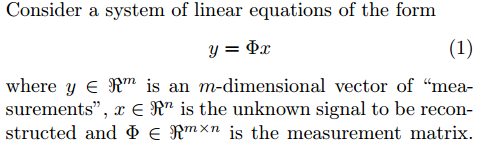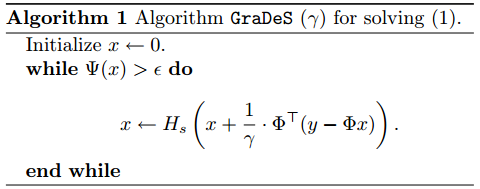其中：
函数Ψ(x)定义如下：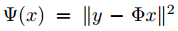符号Hs(·)的含义如下：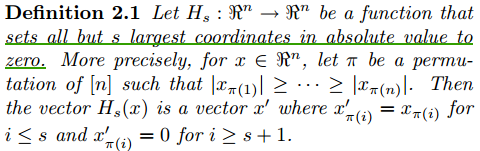也就是说，Hs(x)是将一个n维向量x中最大的s个值保留，其余全部置零，也就是将n维向量x强制变为s稀疏的。文章中紧跟表格Algorithm1有一段有关Hs(·)的描述：参数γ的取值参见Theorem 2.1：即若要恢复一个s稀疏的向量x，γ等于1加上矩阵Φ的2s阶有限等距常数δ2s。另外，在Theorem2.1中要求矩阵Φ的2s阶有限等距常数δ2s<1/3，还给出了具体的迭代次数公式。但个人感觉，Theorem 2.1的结果并没有实际意义，无论是参数γ还是迭代次数，原因很简单，因为在实际当中矩阵Φ的2s阶有限等距常数δ2s根本无法得到。

function [ x ] = GraDeS_Basic( y,Phi,s,gama,epsilon,loopmax )
%GraDeS_Basic Summary of this function goes here
%Version: 1.0 written by jbb0523 @2016-07-28
%Reference:Garg R, Khandekar R. Gradient descent with sparsification: an iterative
%algorithm for sparse recovery with restricted isometry property[C]//Proceedings of
%the 26th Annual International Conference on Machine Learning. ACM, 2009: 337-344
%Available at:http://people.cse.iitd.ernet.in/~rohitk/research/sparse.pdf
%   Detailed explanation goes here
if nargin < 6
loopmax = 3000;
end
if nargin < 5
epsilon = 1e-3;
end
if nargin < 4
gama = 1+1/3;
end
[y_rows,y_columns] = size(y);
if y_rows<y_columns
y = y';%y should be a column vector
end
n = size(Phi,2);
x = zeros(n,1);%Initialize x=0
loop = 0;
while(norm(y-Phi*x)>epsilon && loop < loopmax)
x = x + Phi'*(y-Phi*x)/gama;%update x
%the following two lines of code realize functionality of Hs(.)
%1st: permute absolute value of x in descending order
[xsorted inds] = sort(abs(x), 'descend');
%2nd: set all but s largest coordinates to zeros
x(inds(s+1:n)) = 0;
loop = loop + 1;
end
end

注：本来就单纯想按照论文的Algorithm 1中的流程写了个简简单单的函数，但在while循环条件里面仍然加入了最大循环次数的限制，这是因为仿真过程中发现程序经常处于死循环状态。
这里也给出作者的官方版本（参见链接【4】）：

function [x i] = grades(y, phi, s, tol, niters, weight)
if (nargin < 6)
weight = 1 + 1/3;
end;
if (nargin < 5)
niters = 3e3;
end;
if (nargin < 4)
tol = 1e-10;
end;
if (nargin < 3)
disp('Error:  too few arguments');
return;
end;

y2 = sum(y.*y);
r = y;
r2 = y2;
r_last = r2 + 1;
n = size(phi, 2);
x = zeros(n, 1);
i = 0;
while ((r2 / y2 > tol) && (i < niters) && (r2 / y2 < 1e5) && (r2 < r_last * (1+0.1)))
x = x + phi' * r / weight;
[xsorted inds] = sort(abs(x), 'descend');
x(inds(s+1:n)) = 0;
r = y - phi * x;
r_last = r2;
r2 = sum(r.*r);
i = i+1;
end;
end

3、单次重构代码

%压缩感知重构算法测试
clear all;close all;clc;
M = 64;%观测值个数
N = 256;%信号x的长度
K = 10;%信号x的稀疏度
Index_K = randperm(N);
x = zeros(N,1);
x(Index_K(1:K)) = 5*randn(K,1);%x为K稀疏的，且位置是随机的
Psi = eye(N);%x本身是稀疏的，定义稀疏矩阵为单位阵x=Psi*theta
Phi = randn(M,N);%测量矩阵为高斯矩阵
Phi = orth(Phi')';
A = Phi * Psi;%传感矩阵
% sigma = 0.005;
% e = sigma*randn(M,1);
% y = Phi * x + e;%得到观测向量y
y = Phi * x;%得到观测向量y
%% 恢复重构信号x
tic
% fprintf('\ni=%d\n',i);
x_r = Psi * theta;% x=Psi * theta
toc
%% 绘图
figure;
plot(x_r,'k.-');%绘出x的恢复信号
hold on;
plot(x,'r');%绘出原信号x
hold off;
legend('Recovery','Original')
fprintf('\n恢复残差：');
norm(x_r-x)%恢复残差   

注1：这里不给出仿真结果了，因为仿真结果不太好，总是无法重构；
注2：Phi必须经过“Phi= orth(Phi')';”处理，否则根本没有重构结果输出。
4、为什么能够重构？
该算法比以往介绍的任何算法都要简单，但为什么执行以下迭代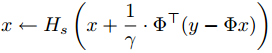最终就可以得到稀疏解x呢？我们来看文章2.1节的一段描述：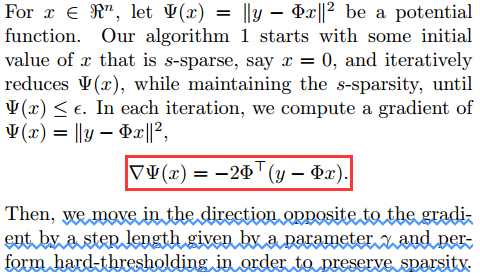也就是说，每次迭代，我们都会根据参数γ沿着目标函数Ψ(x)梯度相反的方向走一步，为了保证每次迭代后仍是s稀疏的，对迭代结果执行硬阈值(hard-thresholding)，也就是函数Hs(·)。
那么这里有两个问题：
一是为什么沿着目标函数Ψ(x)梯度相反的方向走一步会使目标函数变小？
二是执行硬阈值后会不会使目标函数Ψ(x)很大幅度地增加？
对于第一个问题：
实际上，这个类似于最优化方法中的梯度下降法（或称最速下降法），我们举一个简单例子，比如f(x)=x2，求它的梯度（也就是导数）▽f(x)=2x，对于变量x来说只有两个移动方向，要么是正方向，要么是负方向：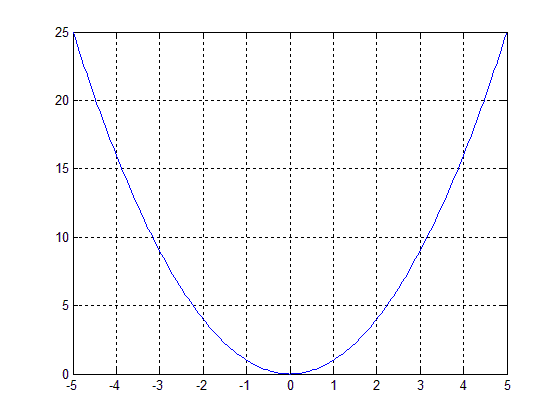假设我们的初始点在x=4，这时梯度▽f(4)=2×4=8>0，梯度方向（单位向量可以通过▽f(x)/|| ▽f(x)||得到）为正方向（即由0指向1），其反方向为负方向（即由0指向-1），从f(x)=x2的图像中可以很容易的知道，从x=4往负方向走一步函数值会下降（当然步子不能迈得太大）；假设我们的初始点在x=-4，这时梯度▽f(4)=2×(-4)=-8<0，梯度方向为负方向（即由0指向-1），其反方向为正方向（即由0指向1），从f(x)=x2的图像中可以很容易的知道，从x=-4往正方向走一步函数值会下降（当然步子也不能迈得太大）。因为这个自变量只有一个，所以可移动的方向只有两个，即在一条直线上移动；如果自变量有两个的话，则移动范围是在一个平面上。求解只有一个变量函数的极值问题即为一维极值问题，求解有多个变量函数的极值问题即为多维极值问题。
对于第二个问题：
为了解释第二个问题，先来看一段文章内容：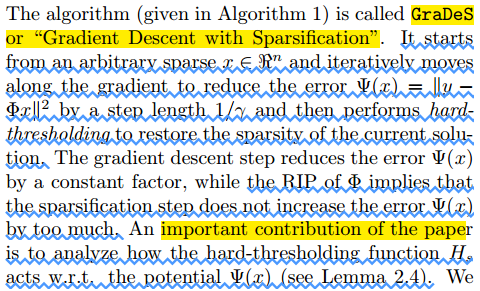注：w.r.t.意思是“关于”，是英文“with regard to”或“withreference to”或“with respect to”的简称。
这段内容首先是对该算法的原理进行了简要的描述，这个类似于分析第一个问题中的引用的那段话；紧跟着有一句非常关键的话“the RIP of Φ impliesthat the sparsification step does not increase the error Ψ(x) by too much”，也就是说Φ的RIP保证了稀疏步骤(sparsificationstep，即执行硬阈值步骤)不会使Ψ(x)误差增加很多。但刨根问底，为什么呢？接下来提到这篇论文重要的贡献是分析了硬阈值函数Hs(·)对Ψ(x)的影响，也就是Lemma 2.4。
引理2.4是由下面内容引出的：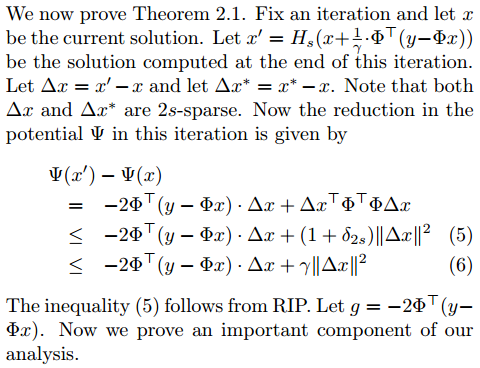来详细推导一下上面的式子：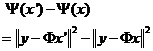其中：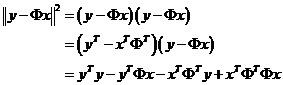同理：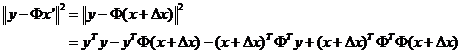将带括号的三项拆开：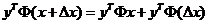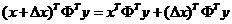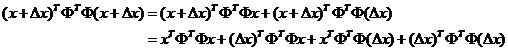所以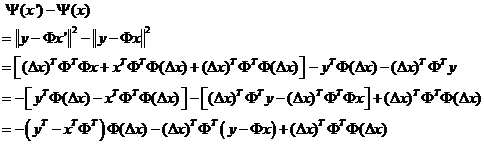由于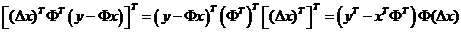所以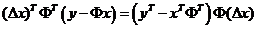注：上面相等的原因是由于二者均为1×1的矩阵，即为一个数；而一个数的共轭等于它本身。
进而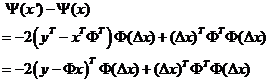值得注意的是文中的推导至此有一点小错误：
矩阵Φ的维数为m×n，因此转置后ΦT的维数为n×m，(y-Φx)的维数是m×1，△x的维数为n×1，所以不可能为文中的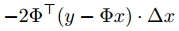因为根本不满足矩阵相乘的维度条件。因此，g的定义应该为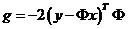式(5)就是应用了RIP性质不等式，这是因为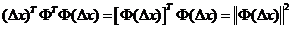根据文中式(3)即可得式(5)。至于这里为什么是2s阶有限等距常数δ2s可简单这样理解：两个s稀疏的向量相减，最坏的情况是两个相量各自s个有值的位置都不对应，所以减完之后的结果最坏情况会包含2s个值，也就是稀疏度是2s。
式(6)就是简单的令γ=δ2s+1便可由式(5)得到（参见定理2.1）。
整个推导结果的意义在于执行一次迭代后，目标函数的变化不大于式(6)。
分析完了引理2.4的铺垫，接下来那么我们就来看一下引理2.4的具体内容：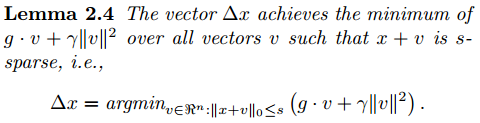引理2.4的意思是，对于目标函数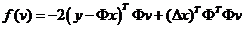在约束条件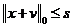即x+v稀疏度不超过s的约束下，其最小值在△x处取得。也就是说式(6)的结果是一个最小值。下面是证明过程，有兴趣的可以研究一下，只要把符号搞清楚也并不是很复杂：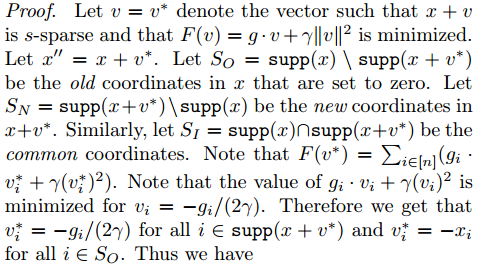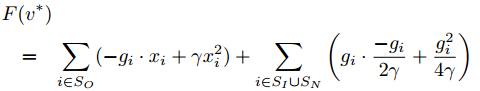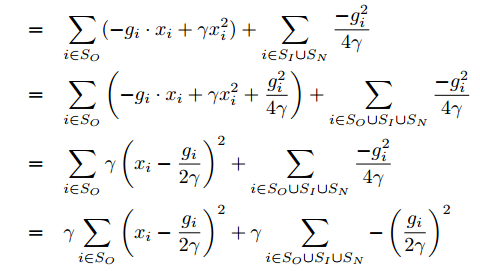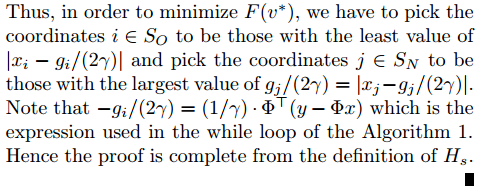5、结束语
另外，学习研究了几天，但重构结果并不是很好，心中有一点失落……
算了，还是继续前行吧……
6、参考链接：
http://www.cse.iitd.ac.in/~rahulgarg/Publications/2009/GK09.icml.pdf
http://people.cse.iitd.ernet.in/~rohitk/research/sparse.pdf
【2】PPT: http://www.cse.iitd.ac.in/~rahulgarg/Publications/2009/GK09.icml.talk.pdf
【3】Author Homepage:
http://www.cse.iitd.ac.in/~rahulgarg/
http://people.cse.iitd.ernet.in/~rohitk/
【4】Code: http://www.cse.iitd.ac.in/~rahulgarg/Code/grades.m
展开全文• 提出了一种基于联合稀疏模型与压缩感知理论的同步子空间追踪算法，以稀疏特性为先验知识，通过回溯迭代方式，判断并选取合适的联合子空间，用更少量观测值实现原始传感数据的精确重构。与SCoSaMP算法、SP算法在不同...无线传感器网络 联合稀疏模型
• # DCT基作为稀疏基，重建算法为OMP算法 ，图像按列进行处理 # 导入所需的第三方库文件 import numpy as np import math from PIL import Image #读取图像，并变成numpy类型的 array im = np.array(Image.open('/...
#coding:utf-8
# DCT基作为稀疏基，重建算法为OMP算法 ，图像按列进行处理
# 导入所需的第三方库文件
import  numpy as np
import math
from PIL import Image

#读取图像，并变成numpy类型的 array
im = np.array(Image.open('/Users/sanfordzhu/Desktop/lena.bmp')) #图片大小256*256

#生成高斯随机测量矩阵
sampleRate=0.5  #采样率
Phi=np.random.randn(int(256*sampleRate),256)

#生成稀疏基DCT矩阵
mat_dct_1d=np.zeros((256,256))
v=range(256)
for k in range(0,256):
dct_1d=np.cos(np.dot(v,k*math.pi/256))
if k>0:
dct_1d=dct_1d-np.mean(dct_1d)
mat_dct_1d[:,k]=dct_1d/np.linalg.norm(dct_1d)

#随机测量
img_cs_1d=np.dot(Phi,im)

#OMP算法函数
def cs_omp(y,D):#传入参数为y和传感矩阵
L=math.floor(y.shape/4)
residual=y  #初始化残差
index=np.zeros((L),dtype=int)
for i in range(L):
index[i]= -1
result=np.zeros((256))
for j in range(L):  #迭代次数
product=np.fabs(np.dot(D.T,residual))
pos=np.argmax(product)  #最大投影系数对应的位置
index[j]=pos
tmp=[]
for tt in range(len(index)):
if (index[tt]>0)or(index[tt]==0):
tmp.append(tt)
tmp1=D[:,tmp]
my = np.linalg.pinv(D[:,tmp])  # 最小二乘
a=np.dot(my,y) #最小二乘
residual=y-np.dot(D[:,tmp],a)
result[tmp]=a
return  result

#重建
sparse_rec_1d=np.zeros((256,256))   # 初始化稀疏系数矩阵
Theta_1d=np.dot(Phi,mat_dct_1d)   #测量矩阵乘上基矩阵
for i in range(256):
print('正在重建第',i,'列。')
column_rec=cs_omp(img_cs_1d[:,i],Theta_1d) #利用OMP算法计算稀疏系数
sparse_rec_1d[:,i]=column_rec;
img_rec=np.dot(mat_dct_1d,sparse_rec_1d)          #稀疏系数乘上基矩阵

#显示重建后的图片
image2=Image.fromarray(img_rec)
image2.show()

展开全文• 时域信号压缩传感,正交匹配追踪法（OMP）重构信号,注释比较详细
• 题目：压缩感知重构算法之正交匹配追踪(OMP)  前面经过几篇的基础铺垫，本篇给出正交匹配追踪(OMP)算法的MATLAB函数代码，并且给出单次测试例程代码、测量数M与重构成功概率关系曲线绘制例程代码、信号稀疏度K与...
• Needell继ROMP之后提出的又一个具有较大影响力的重构算法。CoSaMP也是对OMP的一种改进，每次迭代选择多个原子，除了原子的选择标准之外，它有一点不同于ROMP：ROMP每次迭代已经选择的原子会一直保留，而CoSaMP每次...
• 压缩感知之OMP恢复算法 1、基本思想 　y=Φx x=Ψθ 　正交匹配追踪算法的本质思想是，以贪婪迭代的方式选择测量矩阵Φ的列，使得在每次迭代中所选择的列与当前的冗余向量最大程度地相关，从测量向量中减去相关...
• 如果掌握了压缩采样匹配追踪(CoSaMP)后，再去学习子空间追踪(Subspace Pursuit)是一件非常简单的事情，因为它们几乎是完全一样的。 SP的提出时间比CoSaMP提出时间略晚，首个论文版本是参考文献，后来更新了两次...
•研究论文
• 题目：压缩感知重构算法之基追踪(Basis Pursuit, BP)  除匹配追踪类贪婪迭代算法之外，压缩感知重构算法另一大类就是凸优化算法或最优化逼近方法，这类方法通过将非凸问题转化为凸问题求解找到信号的逼近，其中最...
• 分段正交匹配追踪(StagewiseOMP)或者翻译为逐步正交匹配追踪，它是OMP另一种改进算法，每次迭代可以选择多个原子。此算法的输入参数中没有信号稀疏度K，因此相比于ROMP及CoSaMP有独到的优势。尽管StOMP输入参数中不...
• 浅谈压缩感知（二十七）：压缩感知重构算法之稀疏度自适应匹配追踪(SAMP) 主要内容： SAMP的算法流程SAMP的MATLAB实现一维信号的实验与结果稀疏度K与重构成功概率关系的实验与结果 一、SAMP的算法流程 前面所述...
• 针对光纤布拉格光栅(FBG)传感系统中数据量庞大、不利于数据传输及存储的问题，提出了一种分段自适应采样压缩感知与改进的正交匹配追踪（SASCS-IOMP）算法。利用设计特定参数的Gabor滤波器提取FBG光谱信号上边带斜率...
• 压缩感知重构算法之分段正交匹配追踪(StOMP) 转载自彬彬有礼的的专栏  题目：压缩感知重构算法之分段正交匹配追踪(StOMP)  分段正交匹配追踪(StagewiseOMP)或者翻译为逐步正交匹配追踪，它是OMP另一种改进算法...
• 压缩感知重构算法之广义正交匹配追踪(gOMP) 转载自彬彬有礼的专栏 题目：压缩感知重构算法之广义正交匹配追踪(gOMP)  广义正交匹配追踪(Generalized OMP, gOMP)算法可以看作为OMP算法的一...
• 压缩传感（CS）是利用图像具有稀疏性表示的先验知识，由投影获得的少量观测值来进行图像重建。利用基于CS的联合代数迭代算法进行了太赫兹CT图像仿真重构研究，实验结果验证了算法重构效果以及可用于快速成像的能力......